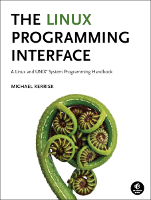procexec/execlp.c

This is procexec/execlp.c, an example to accompany the book, The Linux Programming Interface.

This file is not printed in the book; it is the solution to Exercise 27-2 (page 589).

In the listing below, the names of Linux system calls and C library functions are hyperlinked to manual pages from the Linux man-pages project, and the names of functions implemented in the book are hyperlinked to the implementations of those functions.• Distribution version

Function list (Bold in this list means a function is not static)

/* execlp.c

An implementation of execlp() using execve().

For the full details, see the SUSv3 specification of the exec functions
(XSH:exec). For an alternate (and more complete) implementation, see the
posix/exec*.c sources in glibc.
*/

#include <stdio.h>
#include <stdlib.h>
#include <errno.h>
#include <stdarg.h>
#include <string.h>
#include <unistd.h>
#include <stdbool.h>

extern char **environ;

#define max(x,y) ((x) > (y) ? (x) : (y))
#define SHELL_PATH "/bin/sh"            /* Pathname for the standard shell */
/* Exec a script file using the standard shell */

static void
execShScript(int argc, char *argv[], char *envp[])
{
char *shArgv[argc + 1];

shArgv = SHELL_PATH;
for (int j = 0; j <= argc; j++)
shArgv[j + 1] = argv[j];
execve(SHELL_PATH, shArgv, envp);

/* We only get here if execve() fails, in which case we return
to our caller */
}
int
execlp(const char *filename, const char *arg, ...)
{
/***** Build argument vector from variable-length argument list *****/

int argvSize = 100;         /* Currently allocated size of argv */
char **argv = calloc(argvSize, sizeof(void *));
if (argv == NULL)
return -1;

argv = (char *) arg;
int argc = 1;

/* Walk through variable-length argument list until NULL terminator
is found, building argv as we go */

va_list argList;            /* For variable argument list parsing */
va_start(argList, arg);
while (argv[argc - 1] != NULL) {
if (argc + 1 >= argvSize) {     /* Resize argv if required */
char **nargv;

argvSize += 100;
nargv = realloc(argv, sizeof(void *));
if (nargv == NULL) {
free(argv);
return -1;
} else {
argv = nargv;
}
}

argv[argc] = va_arg(argList, char *);
argc++;
}

va_end(argList);

/***** Use caller's environment to create envp vector *****/

int j;

for (j = 0; environ[j] != NULL; )   /* Calculate size of environ */
j++;
char **envp = calloc(sizeof(void *), j + 1);
if (envp == NULL) {
free(argv);
return -1;
}

for (j = 0; environ[j] != NULL; j++)    /* Duplicate environ in envp */
envp[j] = strdup(environ[j]);
envp[j] = NULL;             /* List must be terminated by NULL pointer */

/***** Now try to exec filename *****/

int fndEACCES = false;      /* True if any execve() returned EACCES */
int savedErrno;

if (strchr(filename, '/') != NULL) {

/* If file contains a slash, it's a pathname and we don't do
a search using PATH */

char *pathname = strdup(filename);

execve(pathname, argv, envp);

savedErrno = errno;             /* So we can return correct errno */
if (errno == ENOEXEC)
execShScript(argc, argv, envp);

free(pathname);                 /* Avoid memory leaks */

} else {            /* Use PATH */

/* Treat undefined PATH as "." */

char *p = getenv("PATH");
char *PATH = (p == NULL || strlen(p) == 0) ? strdup(".") : strdup(p);

/* For each prefix in PATH, try to exec 'filename' in that
directory. The loop will terminate either when we successfully
exec or when we run out of path prefixes. */

char *prStart, *prEnd;  /* Start and end of prefix currently
being processed from PATH */
prStart = PATH;
bool morePrefixes = true;  /* True if there are more prefixes in PATH */

while (morePrefixes) {

/* Locate end of prefix */

prEnd = strchr(prStart, ':');
if (prEnd == NULL)          /* Handle last prefix */
prEnd = prStart + strlen(prStart);

/* Build complete pathname from path prefix and filename */

char *pathname;             /* Path prefix + '/' + filename */
pathname = malloc(max(1, prEnd - prStart) + strlen(filename) + 2);
pathname = '\0';
if (prEnd == prStart)       /* Last prefix */
strcat(pathname, ".");
else
strncat(pathname, prStart, prEnd - prStart);
strcat(pathname, "/");
strcat(pathname, filename);

if (*prEnd == '\0')         /* No more prefixes */
morePrefixes = false;
else
prStart = prEnd + 1;    /* Move to start of next prefix */

/* Try to exec pathname; execve() returns only if we failed */

execve(pathname, argv, envp);
savedErrno = errno;         /* So we can return correct errno */
if (errno == EACCES)
fndEACCES = true;
else if (errno == ENOEXEC)
execShScript(argc, argv, envp);

/* Avoid memory leaks, in case no execve() succeeds! */

free(pathname);
}

free(PATH);
}

/* If we get here, execve() failed; clean up and return -1, ensuring
that errno contains the value returned by (the last) execve() */

free(argv);
for (int j = 0; envp[j] != NULL; j++)
free(envp[j]);
free(envp);

/* SUSv3 says that if any execve() failed with EACCES, then that
is the error that should be returned by exec[lv]p() */

errno = fndEACCES ? EACCES : savedErrno;
return -1;
}

Note that, in most cases, the programs rendered in these web pages are not free standing: you'll typically also need a few other source files (mostly in the lib/ subdirectory) as well. Generally, it's easier to just download the entire source tarball and build the programs with make(1). By hovering your mouse over the various hyperlinked include files and function calls above, you can see which other source files this file depends on.